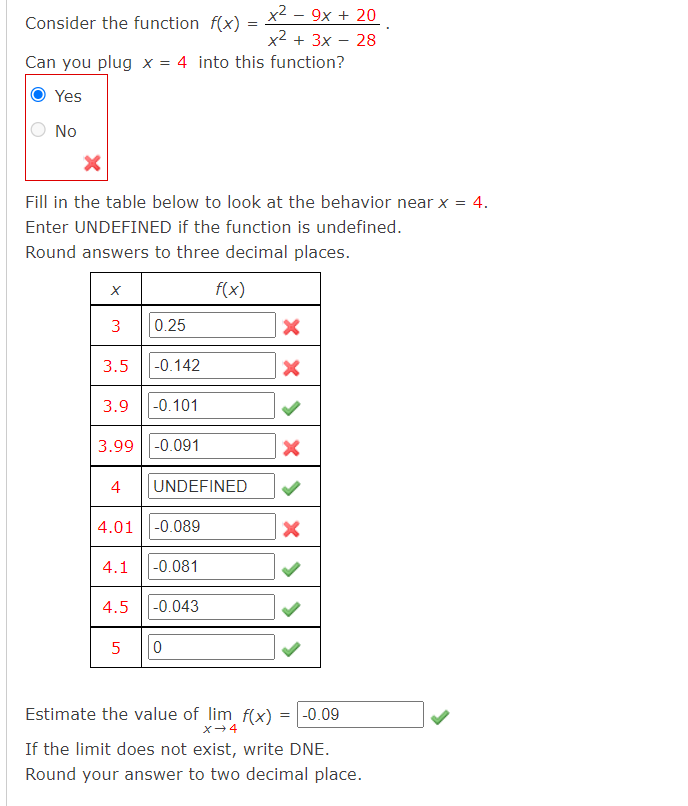Home / Expert Answers / Calculus / consider-the-function-f-x-frac-x-2-9-x-20-x-2-3-x-28-can-you-plug-x-4-into-th-pa162

# (Solved): Consider the function $$f(x)=\frac{x^{2}-9 x+20}{x^{2}+3 x-28}$$. Can you plug $$x=4$$ into th ...Consider the function $$f(x)=\frac{x^{2}-9 x+20}{x^{2}+3 x-28}$$. Can you plug $$x=4$$ into this function? Fill in the table below to look at the behavior near $$x=4$$. Enter UNDEFINED if the function is undefined. Round answers to three decimal places. Estimate the value of $$\lim _{x \rightarrow 4} f(x)=$$ If the limit does not exist, write DNE. Round your answer to two decimal place.

We have an Answer from Expert